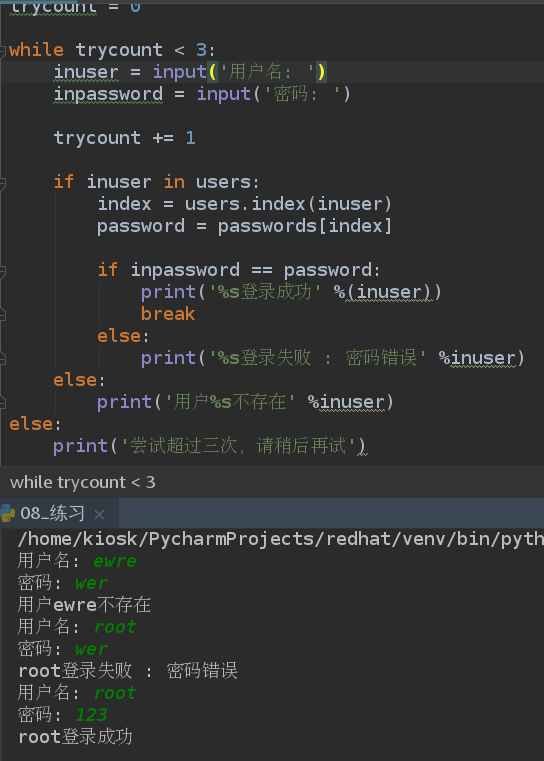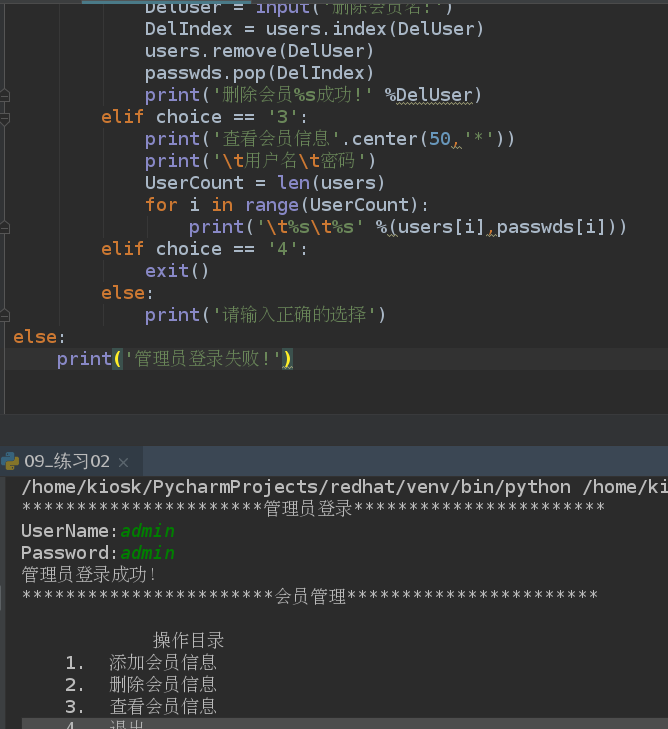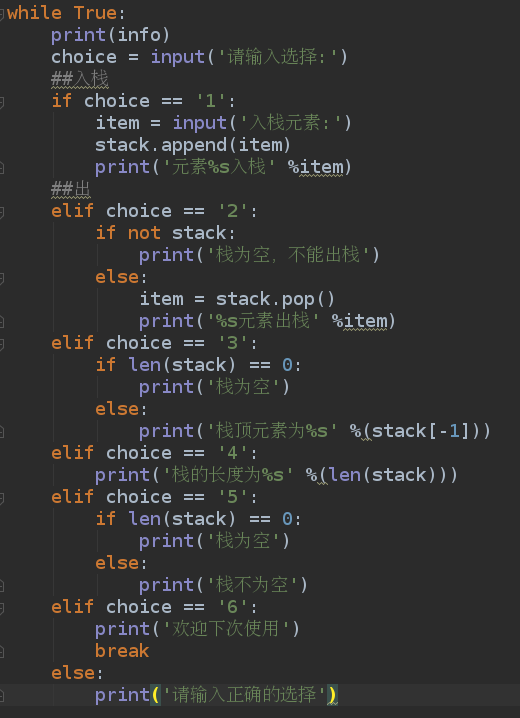## 1.用户登陆系统要求：

“”"
1.系统里面有多个用户，用户的信息目前保存在列表里面
users = [‘root’,‘redhat’]
passwd = [‘123’,‘456’]
2.用户登陆(判断用户登陆是否成功
1).判断用户是否存在
2).如果存在
1).判断用户密码是否正确

3).如果用户不存在

“”"

``````users = ['root','westos']

#尝试登录次数
trycount = 0

while trycount < 3:
inuser = input('用户名: ')
inpassword = input('密码: ')

trycount += 1

if inuser in users:
index = users.index(inuser)

print('%s登录成功' %(inuser))
break
else:
print('%s登录失败 : 密码错误' %inuser)
else:
print('用户%s不存在' %inuser)
else:
print('尝试超过三次，请稍后再试')
``````## 2.管理员用户管理系统要求：

“”"
#2. 当管理员登陆成功后， 可以管理前台会员信息.
#3. 会员信息管理包含:

• 添加会员信息

• 删除会员信息

• 查看会员信息

• 退出

• 添加用户:
1). 判断用户是否存在?
2). 如果存在， 报错；
3). 如果不存在，添加用户名和密码分别到列表中;

• 删除用户
1). 判断用户名是否存在
2). 如果存在，删除；
3). 如果不存在， 报错；
“”"
实现：

``````print('管理员登录'.center(50,'*'))	##美化管理系统

#所有会员用户名
users = ['root','westos']
#所有会员密码
passwds = ['123','456']

if inuser == 'admin' and inpasswd == 'admin':
print('管理员登录成功！')
print('会员管理'.center(50,'*'))
while True:				##写一个循环
print("""			##列出选项
操作目录
1.  添加会员信息
2.  删除会员信息
3.  查看会员信息
4.  退出
""")
choice = input('请选择你的操作:')
if choice == '1':
print('添加会员信息'.center(50,'*'))
if AddUser in users:
else:
elif choice == '2':
print('删除会员信息'.center(50,'*'))
DelUser = input('删除会员名:')
DelIndex = users.index(DelUser)
users.remove(DelUser)
passwds.pop(DelIndex)
print('删除会员%s成功!' %DelUser)
elif choice == '3':
print('查看会员信息'.center(50,'*'))
print('\t用户名\t密码')
UserCount = len(users)
for i in range(UserCount):
print('\t%s\t%s' %(users[i],passwds[i]))
elif choice == '4':
exit()
else:
print('请输入正确的选择')
else:
print('管理员登录失败!')
``````## 3.模拟入栈出栈要求

``````stack = []
info = """
栈操作
1.入栈
2.出栈
3.栈顶元素
4.栈的长度
5.栈是否为空
6.退出
"""
while True:
print(info)
choice = input('请输入选择:')
##入栈
if choice == '1':
item = input('入栈元素:')
stack.append(item)
print('元素%s入栈' %item)
##出栈
elif choice == '2':
if not stack:
print('栈为空，不能出栈')
else:
item = stack.pop()
print('%s元素出栈' %item)
elif choice == '3':
if len(stack) == 0:
print('栈为空')
else:
print('栈顶元素为%s' %(stack[-1]))
elif choice == '4':
print('栈的长度为%s' %(len(stack)))
elif choice == '5':
if len(stack) == 0:
print('栈为空')
else:
print('栈不为空')
elif choice == '6':
print('欢迎下次使用')
break
else:
print('请输入正确的选择')
``````### preg_replace正则表达式的使用_weixin_30951231的博客-程序员宅基地

&lt;?php\$str="as2223adfsf0s4df0sdfsdf";echo preg_replace("/0/","",\$str);//去掉0字符，此时相当于 replace的功能, preg_replace("/0/","A",\$str); 这样就是将0变成A的意思了echo preg_replace("/[0-9]/","",\$str);//去掉所有数字echo p...

### vue+element-ui el-tabs 标签实现在同一页面切换组件_辉＠_＠的博客-程序员宅基地

&lt;template&gt; &lt;div class="main-container"&gt; &lt;el-tabs v-model="currentView"&gt; &lt;el-tab-pane label="电视" name="Television"&gt;&lt;/el-tab-pane&gt; &lt;el-tab-pane label="电影" name="Movie"&gt;&lt;/el-tab-pane&gt; &lt;el-ta